GMTC全球大前端技术大会限时9折特惠中，点击立减¥480 了解详情

# OCR 模型训练

2021 年 1 月 08 日OCR 从流程上包括两步：文本检测文本识别，即将图片输入到文本检测算法中得到一个个的文本框，将每个文本框分别送入到文本识别算法中得到识别结果。

1. 基于深度学习的文本检测算法大致分为两类：基于候选框回归的算法*和*基于分割的算法。

• 基于候选框回归的文本检测，是源于目标检测算法，然后结合文本框的特点改造而成的，包括 CTPN、EAST 和 Seglink 算法等。CTPN 是基于 faster RCNN 改进的算法，在 CNN 后加入 RNN 网络，主要思想是把文本行切分成小的细长矩形进行检测再拼接起来；SegLink 算法的检测思路与 CTPN 类似，也是先检测文本行的小块然后拼起来，但网络结构上采取了 SSD 的思路，在多个特征图尺度上进行文本检测，然后将多尺度的结果融合起来，另外输出中加入了角度信息的回归；EAST 算法，它是直接回归的整个文本行的坐标，而不是细长矩形拼接，网络结构上利用了 Unet 的上采样结构来提取特征，融入了浅层和深层的信息，并且在输出层回归了角度信息，可以检测斜框。

• 基于分割的文本检测，其基本思路是通过分割网络进行像素级别的语义分割，再基于分割的结果构建文本行，包括 PixelLink、Psenet 和 Craft 算法等。PixelLink 算法，网络结构上采用 FCN 提取特征，直接通过实例分割结果中提取文本位置，输出的特征图包括像素分类特征图和像素 link 特征图。Psenet 算法，网络结构上采用 FPN 特征金字塔提取特征，对每个分割区域预测出多个分割结果，然后提出一种新颖的渐进扩展算法，将多个分割的结果进行融合。Craft 算法，网络结构上采用 UNet 的结构，输出的特征图包括 Region score 特征图和像素 Affinity score 特征图，另外特征图中使用了高斯函数，将预测像素点分类的问题转成了像素点的回归问题，能更好的适应文字没有严格包围边界的特点。

2. 基于深度学习的文本识别算法则相对较为统一，一般都采用 CNN+RNN+CTC 的结构，俗称 CRNN 结构，因为这种结构的识别效果很好，且泛化性好，工业上大多都用的这种结构，然后在该框架上做一些改进，如更换 CNN 主干网络，缩减卷积层以提高速度缩减空间，或者改进 RNN 加入 Attention 结构等。

1. 准备训练数据，有的是需要标注（如文本检测中），有的主要是造数据（如文本识别中）；

2. 定义算法网络，这里主要是明确输入和输出；

3. 准备好 batch 数据集，这里主要是处理输入的图片和标签数据，标签数据结构与第 2 步中的网络输出对应，例如 craft 要进行高斯函数计算等，而文本识别中则无需处理，直接将造好的数据输入即可；

4. 定义 loss，优化器和学习率等参数；

5. 训练，这里主要是定义每批次数据训练的操作策略，如保存策略，日志策略，测试策略等。

## OCR 文本检测

### 1、训练数据标注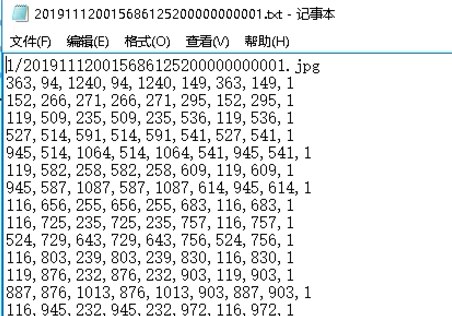### 2、网络设计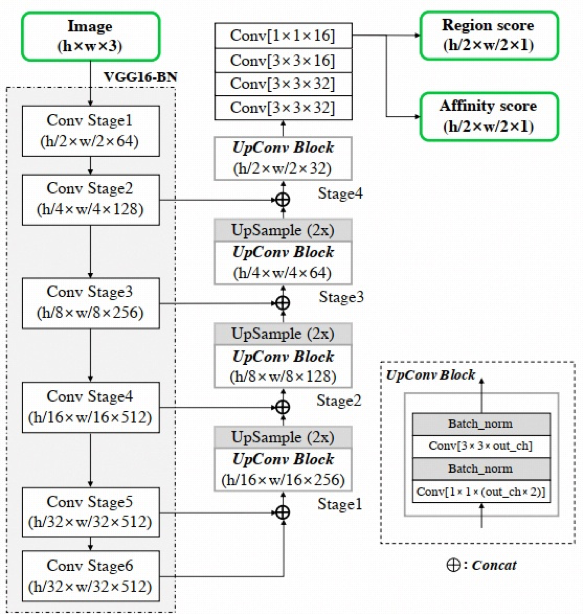class CRAFT(nn.Module):    def __init__(self, pretrained=False, freeze=False, phase='test'):        super(CRAFT, self).__init__()        """ Base network """        self.basenet = vgg16_bn(pretrained, freeze)        """ 固定部分参数，用于迁移学习"""        if phase == 'train':            for p in self.parameters():                p.requires_grad=False        """ U network """        self.upconv1 = double_conv(1024, 512, 256)        self.upconv2 = double_conv(512, 256, 128)        self.upconv3 = double_conv(256, 128, 64)        self.upconv4 = double_conv(128, 64, 32)        num_class = 2        self.conv_cls = nn.Sequential(            nn.Conv2d(32, 32, kernel_size=3, padding=1), nn.ReLU(inplace=True),            nn.Conv2d(32, 32, kernel_size=3, padding=1), nn.ReLU(inplace=True),            nn.Conv2d(32, 16, kernel_size=3, padding=1), nn.ReLU(inplace=True),            nn.Conv2d(16, 16, kernel_size=1), nn.ReLU(inplace=True),            nn.Conv2d(16, num_class, kernel_size=1),        )        init_weights(self.upconv1.modules())        init_weights(self.upconv2.modules())        init_weights(self.upconv3.modules())        init_weights(self.upconv4.modules())        init_weights(self.conv_cls.modules())    def forward(self, x):        """ Base network """        sources = self.basenet(x)        """ U network """        y = torch.cat([sources, sources], dim=1)        y = self.upconv1(y)        y = F.interpolate(y, size=sources.size()[2:], mode='bilinear', align_corners=False)        y = torch.cat([y, sources], dim=1)        y = self.upconv2(y)        y = F.interpolate(y, size=sources.size()[2:], mode='bilinear', align_corners=False)        y = torch.cat([y, sources], dim=1)        y = self.upconv3(y)        y = F.interpolate(y, size=sources.size()[2:], mode='bilinear', align_corners=False)        y = torch.cat([y, sources], dim=1)        feature = self.upconv4(y)        y = self.conv_cls(feature)        return y.permute(0,2,3,1), feature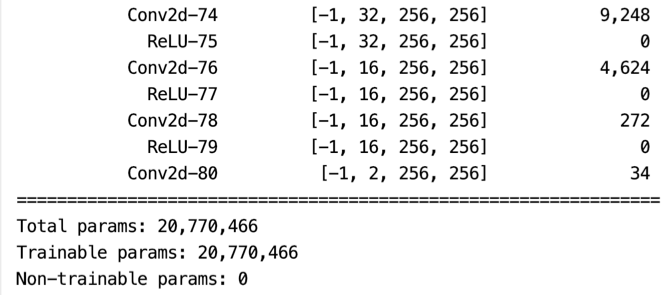### 3、训练标签生成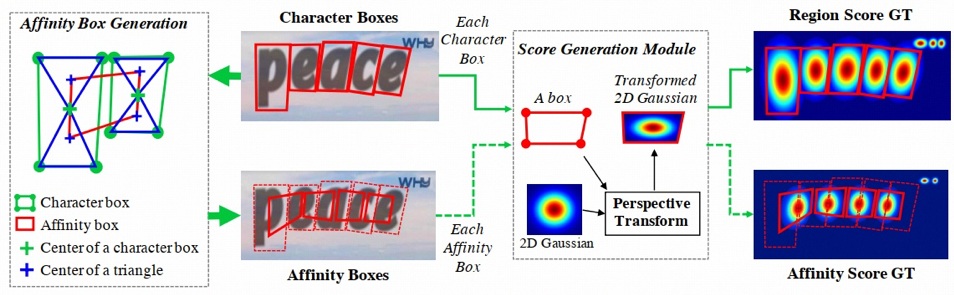def generate_transformed_gaussian_kernel(h, w, points):    '''    使用透视变换的高斯核建模region或affinity    h：图像的高    w：图像的宽    points：维度(4,2)    '''    # 生成高斯核    minX, minY = points    maxX, maxY = points    for i in range(1,4):        minX = min(points[i],minX)        minY = min(points[i],minY)        maxX = max(points[i],maxX)        maxY = max(points[i],maxY)    kernel_w = int((maxX - minX + 1) // 2 * 2)    kernel_h = int((maxY - minY + 1) // 2 * 2)    kernel_size = 31    kernel = np.zeros((kernel_size, kernel_size))    kernel[kernel_size//2, kernel_size//2] = 1    kernel = gaussian_filter(kernel, 10, mode='constant')    kernel_size = max(kernel_h, kernel_w)    kernel = cv2.resize(kernel,(kernel_size,kernel_size))    # 将高斯核透视变换，坐标(列，行)    src = np.float32([(0,0),(0,kernel_size),(kernel_size,kernel_size),(kernel_size,0)]) # 左上，左下，右下，右上    tgt = np.float32(points)    M = cv2.getPerspectiveTransform(src, tgt)    dst = cv2.warpPerspective(kernel, M, (w,h))    # 转换到[0.001,1]之间    mini = dst[np.where(dst>0)].min()    maxi = dst[np.where(dst>0)].max()    h = 1    l = 0.001 # 与预训练模型的分布保持一致    dst[np.where(dst>0)] = ((h-l)*dst[np.where(dst>0)]-h*mini+l*maxi) / (maxi-mini)    return dst

class MyDataset(Dataset):    def __init__(self, root):        self.root = root        self.imglist = [f.split('.') for f in os.listdir(os.path.join(root, 'img'))]    def __getitem__(self, index):        # read img, region_map, affinity_map        img_path = os.path.join(self.root, 'img', self.imglist[index]+'.jpg')#        img = plt.imread(img_path)        img = np.array(plt.imread(img_path))        region_path = os.path.join(self.root, 'region',                                    self.imglist[index].split('_')+'_region_'                                   +self.imglist[index].split('_')+'.npy')        region_map = np.load(region_path).astype(np.float32)        affinity_path = os.path.join(self.root, 'affinity',                                    self.imglist[index].split('_')+'_affinity_'                                   +self.imglist[index].split('_')+'.npy')        affinity_map = np.load(affinity_path).astype(np.float32)        # 保证图像长和宽是2的倍数        h, w, c = img.shape        if h % 2 != 0 or w % 2 != 0:            h = int(h // 2 * 2)            w = int(w // 2 * 2)            img = cv2.resize(img, (w, h))            region_map = cv2.resize(region_map, (w, h))            affinity_map = cv2.resize(affinity_map, (w, h))        # preprocess        img = normalizeMeanVariance(img)        img = torch.from_numpy(img).permute(2, 0, 1)    # [h, w, c] to [c, h, w]        region_map = cv2.resize(region_map, (w//2, h//2))        region_map = torch.tensor(region_map).unsqueeze(2)        affinity_map = cv2.resize(affinity_map, (w//2, h//2))        affinity_map = torch.tensor(affinity_map).unsqueeze(2)        gt_map = torch.cat((region_map,affinity_map), dim=2)        return {'img':img, 'gt':gt_map}    def __len__(self):        return len(self.imglist)

### 4、损失函数设计

criterion=nn.MSELoss(size_average=False).to(device)optimizer=torch.optim.SGD(filter(lambda p: p.requires_grad, net.parameters()),1e-7,                          momentum=0.95,                          weight_decay=0)

### 5、网络训练设计

if __name__ == '__main__':    """参数设置"""    device = 'cuda' # cpu 或 cuda    dataset_path = './data' # 自己数据集的路径    pretrained_path = './pretrained/craft_mlt_25k.pth' # 预训练模型的存放路径    model_path = './models' # 现在训练的模型要存储的路径    dataset = MyDataset(dataset_path)    loader = DataLoader(dataset, batch_size=1, shuffle=True)    net = CRAFT(phase='train').to(device)    net.load_state_dict(copyStateDict(torch.load(pretrained_path, map_location=device)))    criterion=nn.MSELoss(size_average=False).to(device)    optimizer=torch.optim.SGD(filter(lambda p: p.requires_grad, net.parameters()),1e-7,                              momentum=0.95,                              weight_decay=0)    if not os.path.exists(model_path):        os.mkdir(model_path)    for epoch in range(500):        epoch_loss = 0        for i, data in enumerate(loader):            img = data['img'].to(device)            gt = data['gt'].to(device)            # forward            y, _ = net(img)            loss = criterion(y, gt)            optimizer.zero_grad()            loss.backward()            optimizer.step()            epoch_loss += loss.detach()        print('epoch loss_'+str(epoch),':',epoch_loss/len(loader))        torch.save(net.state_dict(), os.path.join(model_path,str(epoch)+'.pth'))

### 6、测试结果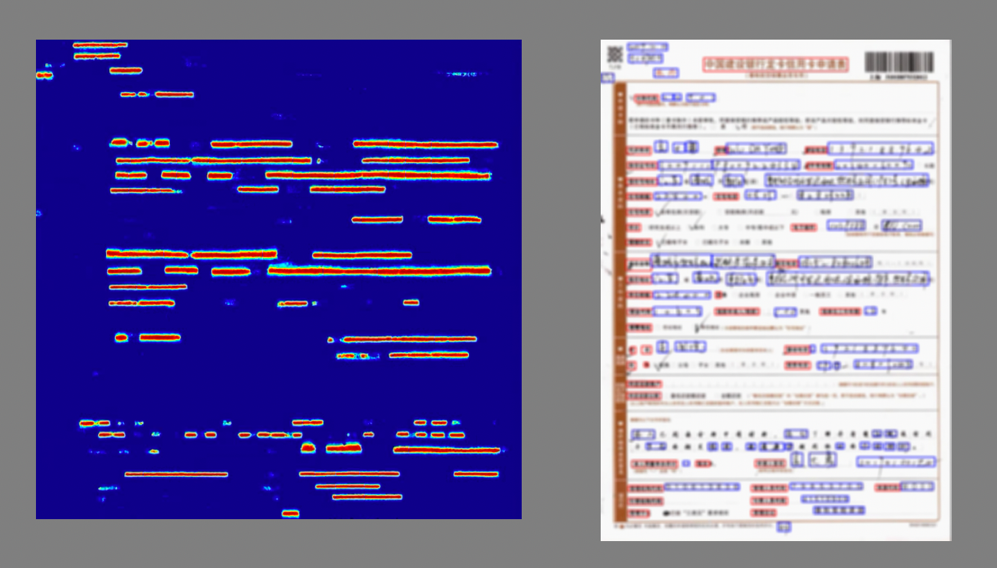## OCR 文本识别

### 1、训练数据生成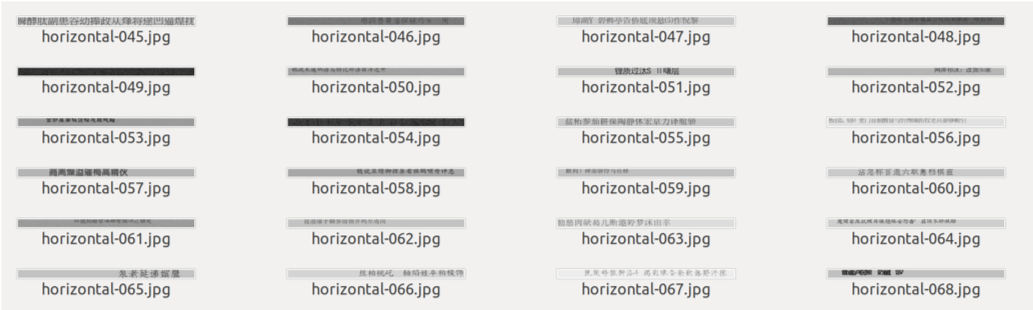OCR 识别模型的训练需要大量的数据，通常需要的数据量是字符集的 1000 倍，例如要训练一个能认 5000 字的模型，至少需要 500 万条数据才能训练，这么多的小图片数据存入内存中，一是占用内存，二是小文件的读取会非常慢。因此在 OCR 识别模型的训练时通常会采用在线的方法。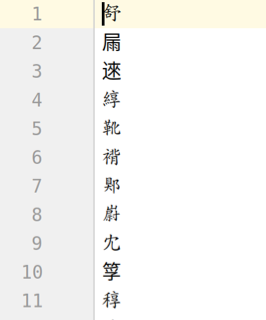char_name = 'chinese_word.txt'data_set = Generator(cfg.word.get_charset(char_name), args.direction, char_name = char_name, word_times = 1000)if args.distributed:    val_sampler = torch.utils.data.distributed.DistributedSampler(val_set)else:    val_sampler = torch.utils.data.RandomSampler(val_set)data_loader = DataLoader(data_set, batch_size=args.batch_size, sampler=train_sampler,                         num_workers=args.workers)class Generator(Dataset):    def __init__(self, alpha, direction='horizontal', char_name = 'chinese_word.txt', word_times = 100):        """        :param alpha: 所有字符        :param direction: 文字方向：horizontal|vertical        """        super(Generator, self).__init__()        self.alpha = alpha        self.direction = direction        self.alpha_list = list(alpha)        self.min_len = 5        self.max_len_list = [16, 19, 24, 26]        self.max_len = max(self.max_len_list)        self.font_size_list = [30, 25, 20, 18]        self.font_path_list = list(FONT_CHARS_DICT.keys())        self.font_list = []  # 二位列表[size,font]        self.word_times = word_times        for size in self.font_size_list:            self.font_list.append([ImageFont.truetype(font_path, size=size)                                   for font_path in self.font_path_list])        if self.direction == 'horizontal':            self.im_h = 32            self.im_w = 512        else:            self.im_h = 512            self.im_w = 32        def get_allchar():            f = codecs.open(os.path.join('./data', char_name),                            mode='r', encoding='utf-8')            lines = f.readlines()            f.close()            charlist = [l.strip() for l in lines]            return charlist        self.charlist = get_allchar()def __getitem__(self, item):    image, indices, target_len = self.gen_image()    if self.direction == 'horizontal':        image = np.transpose(image[:, :, np.newaxis], axes=(2, 1, 0))  # [H,W,C]=>[C,W,H]    else:        image = np.transpose(image[:, :, np.newaxis], axes=(2, 0, 1))  # [H,W,C]=>[C,H,W]    # 标准化    image = image.astype(np.float32) / 255.    image -= 0.5    image /= 0.5    target = np.zeros(shape=(self.max_len,), dtype=np.long)    target[:target_len] = indices    if self.direction == 'horizontal':        input_len = self.im_w // 4 - 3    else:        input_len = self.im_w // 16 - 1    return image, target, input_len, target_lendef __len__(self):return len(self.alpha) * self.word_times

### 2、网络设计

class CRNN(nn.Module):    def __init__(self, num_classes, **kwargs):        super(CRNN, self).__init__(**kwargs)        self.cnn = nn.Sequential(OrderedDict([            ('conv_block_1', _ConvBlock(1, 64)),  # [B,64,W,32]            ('max_pool_1', nn.MaxPool2d(2, 2)),  # [B,64,W/2,16]            ('conv_block_2', _ConvBlock(64, 128)),  # [B,128,W/2,16]            ('max_pool_2', nn.MaxPool2d(2, 2)),  # [B,128,W/4,8]            ('conv_block_3_1', _ConvBlock(128, 256)),  # [B,256,W/4,8]            ('conv_block_3_2', _ConvBlock(256, 256)),  # [B,256,W/4,8]            ('max_pool_3', nn.MaxPool2d((2, 2), (1, 2))),  # [B,256,W/4,4]            ('conv_block_4_1', _ConvBlock(256, 512, bn=True)),  # [B,512,W/4,4]            ('conv_block_4_2', _ConvBlock(512, 512, bn=True)),  # [B,512,W/4,4]            ('max_pool_4', nn.MaxPool2d((2, 2), (1, 2))),  # [B,512,W/4,2]            ('conv_block_5', _ConvBlock(512, 512, kernel_size=2, padding=0))  # [B,512,W/4,1]        ]))        self.rnn1 = nn.GRU(512, 256, batch_first=True, bidirectional=True)        self.rnn2 = nn.GRU(512, 256, batch_first=True, bidirectional=True)        self.transcript = nn.Linear(512, num_classes)def forward(self, x):        """        :param x: [B, 1, W, 32]        :return: [B, W,num_classes]        """        x = self.cnn(x)  # [B,512,W/16,1]        x = torch.squeeze(x, 3)  # [B,512,W]        x = x.permute([0, 2, 1])  # [B,W,512]        x, h1 = self.rnn1(x)        x, h2 = self.rnn2(x, h1)        x = self.transcript(x)        return x

class _ConvBlock(nn.Sequential):    def __init__(self, in_channels, out_channels, kernel_size=3, stride=1, padding=1, bn=False):        super(_ConvBlock, self).__init__()        self.add_module('conv', nn.Conv2d(in_channels, out_channels, kernel_size, stride, padding))        if bn:            self.add_module('norm', nn.BatchNorm2d(out_channels))        self.add_module('relu', nn.ReLU(inplace=True))

### 3、损失函数的设计

model = crnn.CRNN(len(data_set.alpha))model = model.to(device)criterion = CTCLoss()criterion = criterion.to(device)

### 4、网络训练设计

def train_one_epoch(model, criterion, optimizer, data_loader, val_set, device, epoch, args):    model.train()    epoch_loss = 0.0    # for image, target, input_len, target_len in tqdm(data_loader):    for i, sample_batched in enumerate(data_loader):        image, target, input_len, target_len = sample_batched        image = image.to(device)        # print(target, target_len, input_len)        outputs = model(image.to(torch.float32))  # [B,N,C]        outputs = torch.log_softmax(outputs, dim=2)        outputs = outputs.permute([1, 0, 2])  # [N,B,C]        loss = criterion(outputs[:], target, input_len, target_len)        # 梯度更新        model.zero_grad()        loss.backward()        optimizer.step()        # 当前轮的loss        epoch_loss += loss.item() * image.size(0)        # 每训练一个batch打印一次 loss 和 acc        if i % 100 == 0:            print('[epoch:%d, %d | %d] Loss: %.03f'                  % (epoch, i, len(data_loader), epoch_loss / (i + 1)))        if np.isnan(loss.item()):            print(target, input_len, target_len)    epoch_loss = epoch_loss / len(data_loader.dataset)    # 打印日志,保存权重print('Epoch: {}/{} loss: {:03f}'.format(epoch + 1, args.epochs, epoch_loss))

### 5、结果展示

def inference_image(net, alpha, image_path):    image = load_image(image_path)    image = torch.FloatTensor(image)    predict = net(image).detach().numpy()  # [W,num_classes]    label = np.argmax(predict[:], axis=1)    label = [alpha[class_id] for class_id in label]    label = [k for k, g in itertools.groupby(list(label))]    label = ''.join(label).replace(' ', '')return labeldef main(args):    alpha = cfg.word.get_charset('chinese_word.txt')    if args.direction == 'horizontal':        net = crnn.CRNN(num_classes=len(alpha))    else:        net = crnn.CRNNV(num_classes=len(alpha))    net.load_state_dict(torch.load(args.weight_path, map_location='cpu')['model'])    net.eval()    # load image    if args.image_dir:        image_path_list = [os.path.join(args.image_dir, n) for n in os.listdir(args.image_dir)]        image_path_list.sort()        for image_path in image_path_list:            label = inference_image(net, alpha, image_path)            print("image_path:{},label:{}".format(image_path, label))    else:        label = inference_image(net, alpha, args.image_path)        print("image_path:{},label:{}".format(args.image_path, label))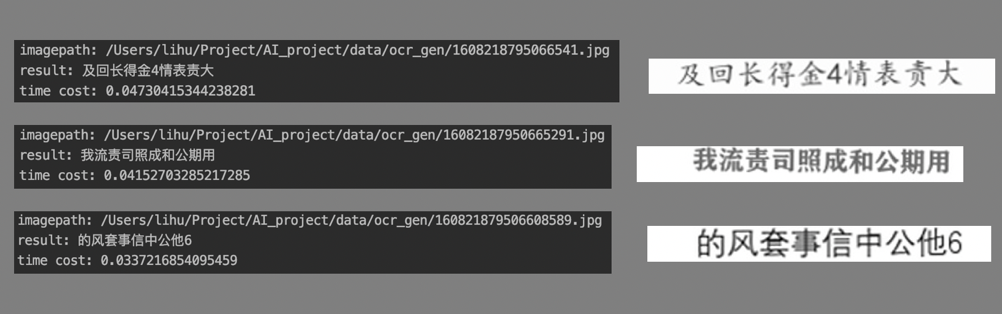2021 年 1 月 08 日 08:001378

## 评论

• ###### 19 人工神经网络 | 各人自扫门前雪：径向基函数神经网络

在神经科学中，什么是“感受野”？如何训练径向基函数神经网络？

2018 年 1 月 20 日

• ###### 计算机视觉领域的深度学习模型（三）：ResNet

今天要跟你分享的这篇论文，获得了CVPR 2016的最佳论文，提出了“残差网络”这个概念。

2018 年 9 月 12 日

• ###### 全局特征与局部特征的交响曲—ParseNet

尽管多尺度融合、空洞卷积都可以增加网络的感受野，但理论感受野与实际感受野仍然存在差异。那么如何真正利用图像的全局信息呢？ParseNet告诉你。

• ###### 苏宁 11.11：一种基于神经网络的智能商品税分类系统

本文的思路主要是根据商品名称分词后生成的词向量，基于神经网络学习一个文本分类模型，在此基础上构建一个智能商品税分类系统。

• ###### 如何应用 MTCNN 和 FaceNet 模型实现人脸检测及识别

随着深度学习的发展，基于深度学习技术的人脸检测 / 识别方法取得了巨大的成功，本文主要介绍人脸检测的深度学习模型 MTCNN 和人脸识别的深度学习模型 FaceNet。

• ###### 结合代码带你理解 DeepFM

本文结合代码详细介绍了DeepFM

• ###### 商汤 ICCV 论文解读：自然场景下文字检测的几何归一化网络（GNNets）

本文通过对待处理图像的特征图进行几何变换，将待处理图像中几何分布差异较大的文本框归一化到一定的几何分布范围内，提高了自然场景下文本测检的效果。

• ###### 基于 Pytorch 的多类别图像分类实战

本篇基于Pytorch完成一个多类别图像分类实战。 实现一个完整的图像分类任务，大致需要分为五个步骤：

2019 年 3 月 4 日

• ###### 27 丨 K-Means（下）：如何使用 K-Means 对图像进行分割？

今天我们继续用K-Means进行聚类的实战。聚类的一个常用场景就是对图像进行分割。

2019 年 2 月 13 日

• ###### Hinton 领衔谷歌大脑新研究，通过胶囊网络重构自动检测对抗样本

胶囊网络（capsule network，CapsNet）可以自动把对抗样本揪出来啦！这项研究来自 capsule 模型的原作者 Sabour、Frosst 以及 Hinton，相关论文将在 NIPS 2018 的机器学习安全研讨会中展示。

• ###### 图形系统如何表示颜色？

前端工程师只知道RGB和RGBA就够了吗？你知道Web图形系统中除了RGB还有哪几种表示颜色的方法吗？

2020 年 7 月 15 日

本文来自华为云开发者社区

• ###### 从感知机到神经网络算法

从机器学习模型角度看，目前最简单的机器学习模型大概就是感知机了，而最火热的机器学习模型则是神经网络。

2019 年 1 月 31 日

• ###### 车道线检测在 AR 导航中的应用与挑战

本文为《高德技术2019年刊合辑》系列之一。

• ###### 无监督学习新突破！华为美研所提出自动编码变换网络 AET

用无监督学习逼近全监督效果

• ###### 人脸关键点定位算法在实际应用中的经验总结

由于深度神经网络强大的特征提取能力，基于深度学习的人脸关键点定位算法在测评指标如NME和FR 上迅速超越传统方法。

rust

#### 浅谈大数据建模的主要技术：维度建模